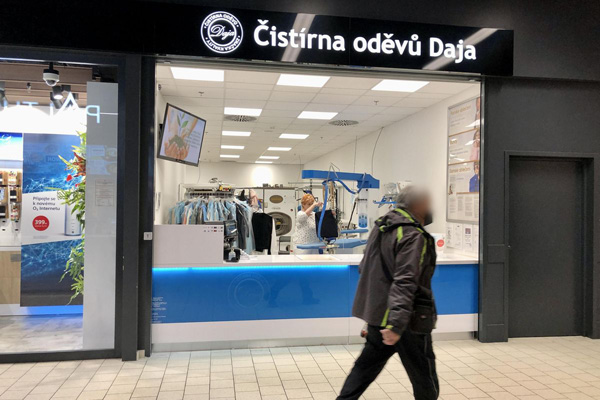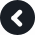Accept

# Dry Cleaning Prague 6

Address: HM Kaufland Vypich, Bělohorská 205, Praha 6

Telephone: +420 235 310 438

Opening hours:
Mon - Sun 8.00 am – 8.00 pm

How to get to us:
Autobus: 164, 168, 174, 180, 184
Tram: 22, 25
Parking available on sitex x x x x x x x x x x x x x x x x x x x x x x x x x x x x x x x x x x x x x x x x x x x x x x x x x x x x x x x x x x x x x x x x x x x x x x x x x x x x x x x x x x x x x x x

## Our Awards